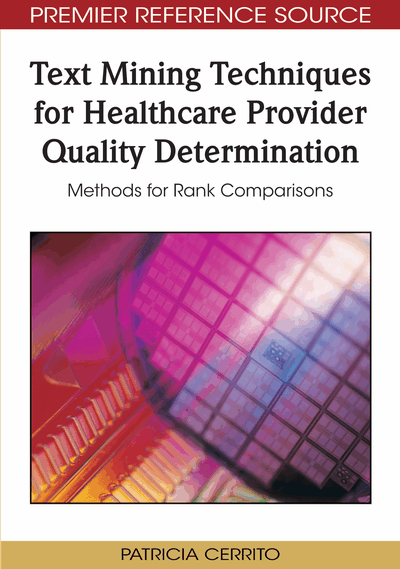# Statistical Methods

Patricia Cerrito (University of Louisville, USA)
DOI: 10.4018/978-1-60566-752-2.ch003
Available
\$37.50
No Current Special Offers

## Abstract

Ultimately, a patient severity index is used to compare patient outcomes across healthcare providers. If the outcome is mortality, logistic regression is used. If the outcome is cost, length of stay, or some other resource utilization, then linear regression is used. A provider is ranked based upon the differential between predicted outcome and actual outcome. The greater this differential, the higher the quality ranking. There are two ways to increase this differential. The first is to improve care to decrease actual mortality or length of stay. The second is to improve coding to increase the predicted mortality or length of stay. Ultimately, it is cheaper to increase the predicted values than it is to decrease the actual values. Many providers take this approach.
Chapter Preview
Top

## Background

Use of the Central Limit Theorem assumes that patients can be treated within 2 standard deviations of the average. However, with a heavy tail, there can be a considerable differential between these two standard deviations and 5-10 percent of the extreme patients.(Battioui, 2007a) One of the reasons for making the assumption of normal distributions is that most of our available statistical models require this assumption. What happens if the assumption of normality is not valid? What happens if the Central Limit Theorem lacks practical meaning? We must be able to work with data in the absence of these assumptions.

According to the Central Limit theorem, the sample mean can be assumed normal if the sample is sufficiently large. If the distribution is exponential, just how large is large enough? We need to examine the requirements of the Central Limit Theorem to explore the concept of large. Also, we want to examine patient-level data rather than group-level data. That will mean that we will want to include information about patient condition in any regression model. A modification of the standard regression, called the generalized linear model can assume population distributions that are not normal. This model can be used to examine gamma and exponential distributions that are often found in medical data.

## Complete Chapter List

Search this Book:
Reset